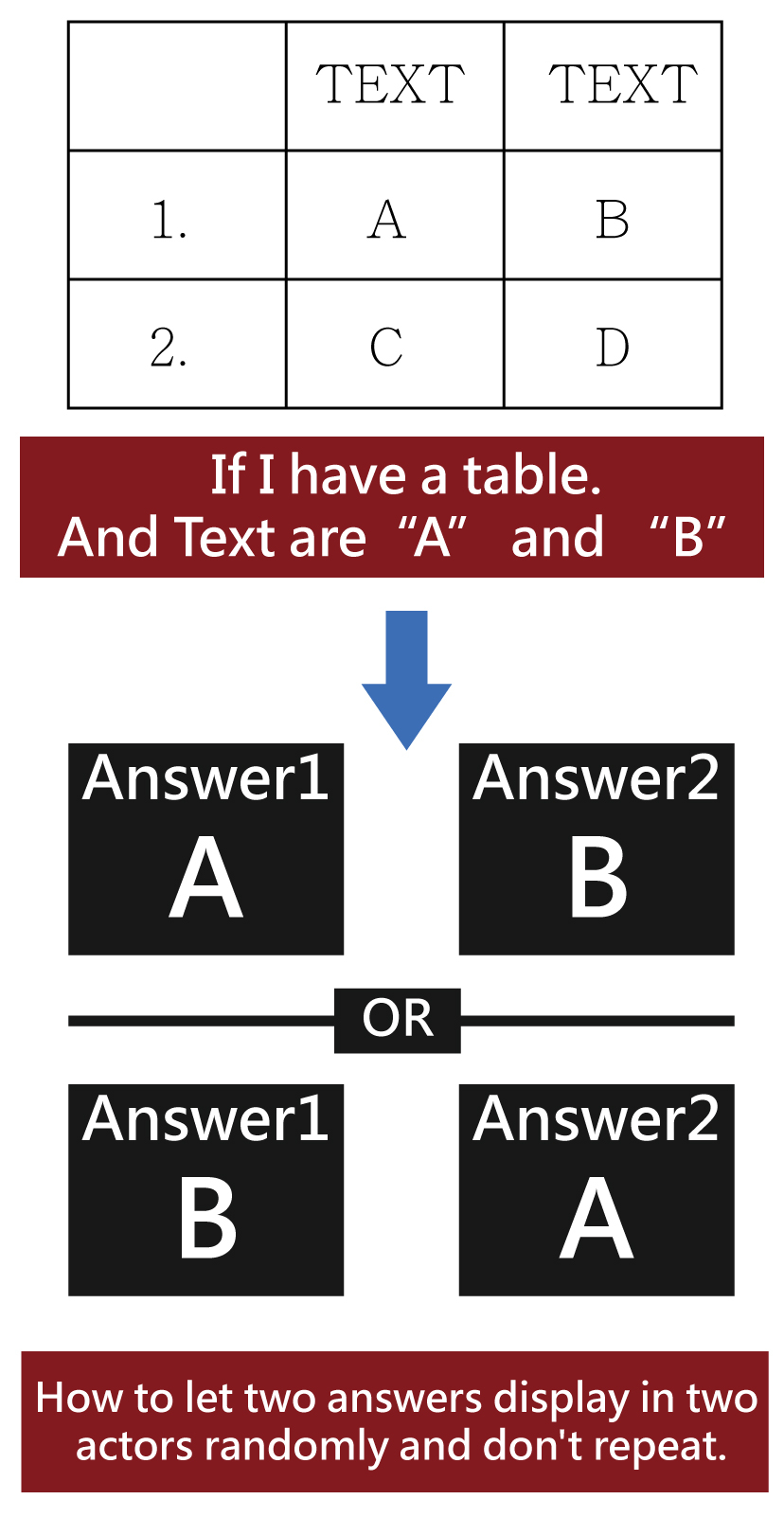# How to let two answers display in two actors randomly and don't repeat.

edited January 2018

Hello everyone! I have a question about table value.

I want to make a "2 choose 1" game.
But I have no idea how to let two answers display in two actors randomly and don't repeat.

For excemple: If Table have two texts which are "A" and "B". At scene, have two actors which display answer1 and answer2.

How to let two answers display in two actors randomly and don't repeat.

Like this:My English is not good, I hope you understand what I said.
Thank you.• Hi @Weiyu,

One way you can do this is to set Answer1 and Answer2 as global attributes.

Then for Actor 1 you can randomly select the first answer by using Change Attribute:-

`game.Answer1 = tableCellValue( game.Table ,1,random(1,2))`

You can do the same to make Actor 2 pick a random table value:-

`game.Answer2 = tableCellValue( game.Table ,1,random(1,2))`

Now, to make sure Actor 2 does not give same answer as Actor 1 use a loop:-

``````While game.Answer1 IS game.Answer2

Change Attribute game.Answer 2 = tableCellValue( game.Table ,1,random(1,2))
``````

Then set a rule to only display text when game.Answer1 IS NOT game.Answer2

Now when you display Answer1 and Answer2 as text they will be random but unique.

I hope that helps!

• Also, you can use mathematically proxy variables.
Then change the displayed variables after the checks.

Not really needed, just one way to stop one of the numbers flickering.